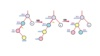avl树 订阅

AVL tree

1962

AVL树

AVL树特点
AVL树本质上还是一棵二叉搜索树，它的特点是：   1.本身首先是一棵二叉搜索树。2.带有平衡条件：每个结点的左右子树的高度之差的绝对值（平衡因子）最多为1。也就是说，AVL树，本质上是带了平衡功能的二叉查找树（二叉排序树，二叉搜索树）。

• ## AVL树

万次阅读 2021-02-08 18:47:14
AVL树的性质 AVL树(Balanced Binary Tree or Height-Balanced Tree) AVL树或者是空二叉树，或者是具有如下性质的BST： 根结点的左、右子树高度之差的绝对值不超过1 且根结点左子树和右子树仍然是AVL树。 结点的...
AVL树的性质
AVL树(Balanced Binary Tree or Height-Balanced Tree)
AVL树或者是空二叉树，或者是具有如下性质的BST：

根结点的左、右子树高度之差的绝对值不超过1
且根结点左子树和右子树仍然是AVL树。

结点的平衡因子BF（Balanced Factor）

一个结点的左子树与右子树的高度之差。
AVL树中的任意结点的BF只可能是-1，0和1。
AVL树的ASL可保持在O(log2n)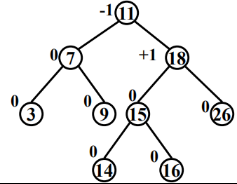在AVL树在结点高度上采用相对平衡的策略，使其平均性能接近于BST的最好情况的性能。
因此，对于包含n个结点的AVL树，其最坏情况下的查找、插入和删除操作时间复杂度均为O(log n)
AVL树的平衡化处理
向AVL树插入结点可能造成不平衡，此时要调整树的结构，使之重新达到平衡
在一棵AVL树上插入结点可能会破坏树的平衡性，需要平衡化处理恢复平衡，且保持BST的结构性质。   若用Y表示新插入的结点，A表示离新插入结点Y最近的，且平衡因子变为±2的祖先结点。
可以用4种旋转进行平衡化处理:

LL型：新结点Y 被插入到 A 的左子树的左子树上（顺）
RR型：新结点Y 被插入到 A 的右子树的右子树上（逆）
LR型：新结点Y 被插入到 A 的左子树的右子树上（逆、顺）
RL型：新结点Y 被插入到 A 的右子树的左子树上（顺、逆）

1.LL型：新结点Y 被插入到 A 的左子树的左子树上（顺）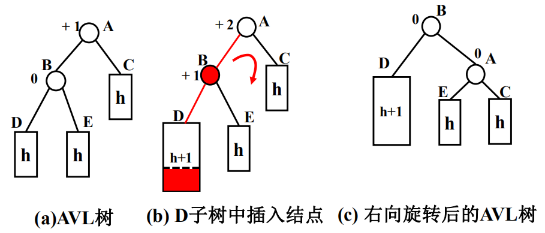2.RR型：新结点Y 被插入到 A 的右子树的右子树上（逆）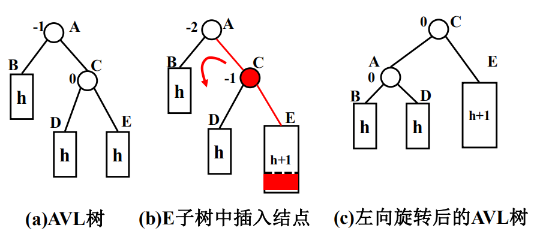LR型：新结点Y 被插入到 A 的左子树的右子树上(逆,顺)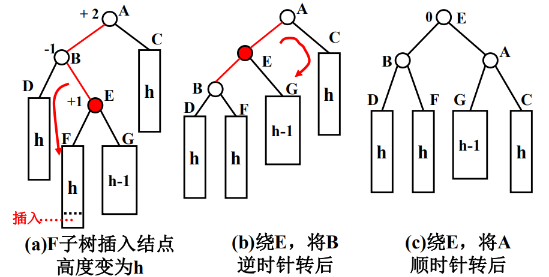RL型：新结点Y 被插入到 A 的右子树的左子树上(顺, 逆)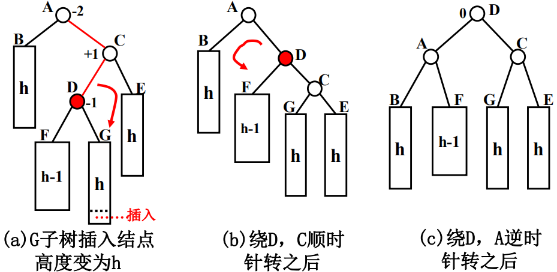平衡调整代码实现：
左旋
  //左旋转方法
private void leftRotate() {

//创建新的结点，以当前根结点的值
Node newNode = new Node(value);
//把新的结点的左子树设置成当前结点的左子树
newNode.left = left;
//把新的结点的右子树设置成带你过去结点的右子树的左子树
newNode.right = right.left;
//把当前结点的值替换成右子结点的值
value = right.value;
//把当前结点的右子树设置成当前结点右子树的右子树
right = right.right;
//把当前结点的左子树(左子结点)设置成新的结点
left = newNode;

}


右旋
 //右旋转
private void rightRotate() {
Node newNode = new Node(value);
newNode.right = right;
newNode.left = left.right;
value = left.value;
left = left.left;
right = newNode;
}

平衡调整
 //平衡调整
private void balanced(){
//当添加或删除完一个结点后，如果: (右子树的高度-左子树的高度) > 1 , 左旋转
if(rightHeight() - leftHeight() > 1) {
//如果它的右子树的左子树的高度大于它的右子树的右子树的高度
if(right != null && right.leftHeight() > right.rightHeight()) {//RL型
//先对右子结点进行右旋转
right.rightRotate();
//然后在对当前结点进行左旋转
leftRotate(); //左旋转..
} else {//RR型
//直接进行左旋转即可
leftRotate();
}
return ; //必须要!!!
}

//当添加完一个结点后，如果 (左子树的高度 - 右子树的高度) > 1, 右旋转
if(leftHeight() - rightHeight() > 1) {
//如果它的左子树的右子树高度大于它的左子树的高度
if(left != null && left.rightHeight() > left.leftHeight()) {//LR型
//先对当前结点的左结点(左子树)->左旋转
left.leftRotate();
//再对当前结点进行右旋转
rightRotate();
} else {//LL型
//直接进行右旋转即可
rightRotate();
}
}

}

AVL树的插入操作与建立

对于一组关键字的输入序列，从空开始不断地插入结点，最后构成AVL树
每插入一个结点后就应判断从该结点到根的路径上有无结点发生不平衡
如有不平衡问题，利用旋转方法进行树的调整，使之平衡化
建AVL树过程是不断插入结点和必要时进行平衡化的过程

示例：
假设 25，27，30，12, 11，18，14，20，15，22 是一关键字序列，并以上述顺序建立AVL树。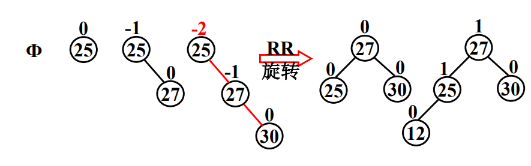示例：假设25，27，30，12， 11，18，14，20，15，22 是一关键字序列，并以上述顺序建立AVL树。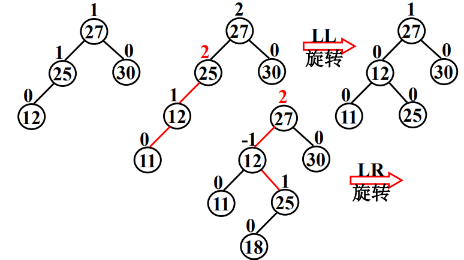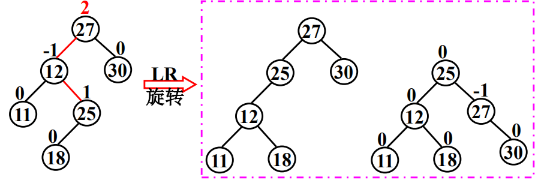示例：假设25，27，30，12，11，18， 14，20，15，22 是一关键字序列，并以上述顺序建立AVL树。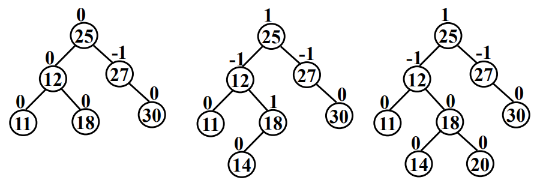示例：假设25，27，30，12，11，18，14，20， 15，22 是一关键字序列，并以上述顺序建立AVL树。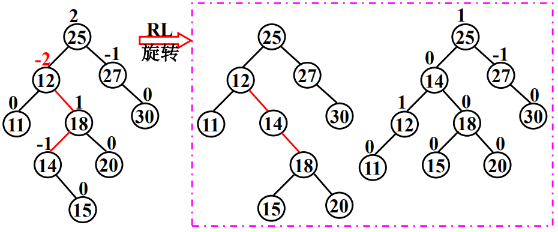示例：假设25，27，30，12，11，18，14，20，15， 22 是一关键字序列，并以上述顺序建立AVL树。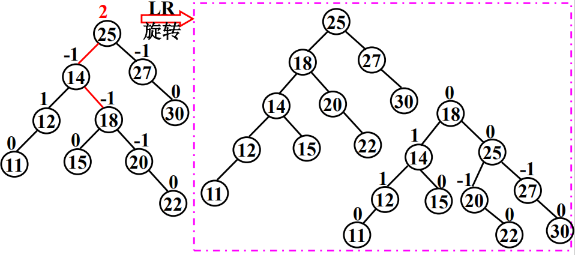插入节点的代码实现：
 // 添加结点的方法
// 递归的形式添加结点，注意需要满足二叉排序树的要求
public void add(Node node) {
if (node == null) {
return;
}

// 判断传入的结点的值，和当前子树的根结点的值关系
if (node.value < this.value) {
// 如果当前结点左子结点为null
if (this.left == null) {
this.left = node;
} else {
// 递归的向左子树添加
this.left.add(node);
}
} else { // 添加的结点的值大于 当前结点的值
if (this.right == null) {
this.right = node;
} else {
// 递归的向右子树添加
this.right.add(node);
}
}
//添加节点之后做平衡调整
balanced();
}

AVL树的删除操作
删除与插入操作是对称的（镜像，互逆的）：

删除右子树结点导致失衡时，相当于在左子树插入导致失衡，即LL或LR；
删除左子树结点导致失衡时，相当于在右子树插入导致失衡，即RR或RL；

删除操作可能需要多次平衡化处理

因为平衡化不会增加子树的高度，但可能会减少子树的高度。
在有可能使树增高的插入操作中，一次平衡化能抵消掉树增高；
而在有可能使树减低的删除操作中，平衡化可能会带来祖先结点的不平衡。
因此，删除操作可能需要多次平衡化处理。

示例：删除15，2次旋转到根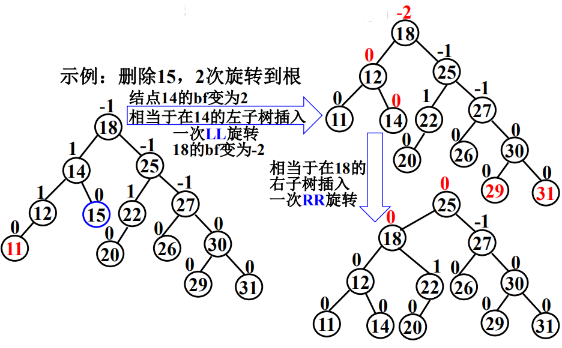AVL树的节点删除原则与BST树(跳转)的原则相同，只需在BST树的基础上做一些修改即可，即在删除节点之后判断是否需要平衡调节即可
删除AVL树的节点代码实现：
 // 编写方法:
// 1. 返回的 以node 为根结点的二叉排序树的最小结点的值
// 2. 删除node 为根结点的二叉排序树的最小结点
/**
*
* @param node
*            传入的结点(当做二叉排序树的根结点)
* @return 返回的 以node 为根结点的二叉排序树的最小结点的值
*/
public int delRightTreeMin(Node node) {
Node target = node;
// 循环的查找左子节点，就会找到最小值
while (target.left != null) {
target = target.left;
}
// 这时 target就指向了最小结点
// 删除最小结点
del(target.value);
return target.value;
}

//删除节点
public void del(int value){

//找不到待删除的节点
if(this.left ==null &&this.right ==null){
System.out.println("未找到待删除节点");
return;
}
// 如果当前结点就是要删除的结点的父结点,或者当前节点就是待删除节点 root
if ((this.left != null && this.left.value == value) || (this.right != null && this.right.value == value) || this.value ==value) {

Node targetNode;//待删除的目标节点
Node parent ;
if(this.value ==value){//当待删除的节点没有父节点，即删除的是root节点，
parent = null;
targetNode =this;
}else {
parent = this;
targetNode = parent.left != null && parent.left.value == value ? parent.left : parent.right;
}

// 如果要删除的结点是叶子结点
if (targetNode.left == null && targetNode.right == null) {
// 判断targetNode 是父结点的左子结点，还是右子结点
if (parent.left != null && parent.left.value == value) { // 是左子结点
parent.left = null;
} else if (parent.right != null && parent.right.value == value) {// 是由子结点
parent.right = null;
}
} else if (targetNode.left != null && targetNode.right != null) { // 删除有两颗子树的节点
int minVal = delRightTreeMin(targetNode.right);
targetNode.value = minVal;

} else { // 删除只有一颗子树的结点
// 如果要删除的结点有左子结点
if (targetNode.left != null) {
if (parent != null) {
// 如果 targetNode 是 parent 的左子结点
if (parent.left.value == value) {
parent.left = targetNode.left;
} else { // targetNode 是 parent 的右子结点
parent.right = targetNode.left;
}
}
} else { // 如果要删除的结点有右子结点
if (parent != null) {
// 如果 targetNode 是 parent 的左子结点
if (parent.left.value == value) {
parent.left = targetNode.right;
} else { // 如果 targetNode 是 parent 的右子结点
parent.right = targetNode.right;
}
}
}

}

}
// 如果查找的值小于当前结点的值, 并且当前结点的左子结点不为空
if (value < this.value && this.left != null) {
this.left.del(value); // 向左子树递归查找
}  // 如果查找的值大于当前结点的值, 并且当前结点的左子结点不为空
if (value >= this.value && this.right != null) {
this.right.del(value); // 向右子树递归查找
}
//删除之后做平衡调整，递归返回时依次从下网上调整
balanced();

}

完整代码(java)
public class AVLTreeDemo {

public static void main(String[] args) {

int[] arr = {25,27,30,12,11,18,14,20,15,22 };
//创建一个 AVLTree对象
AVLTree avlTree = new AVLTree();
//添加结点
for(int i=0; i < arr.length; i++) {
avlTree.add(new Node(arr[i]));
}
//遍历
System.out.println("中序遍历");
avlTree.infixOrder();
System.out.println("在平衡处理~~");
System.out.println("树的高度=" + avlTree.getRoot().height()); //3
System.out.println("树的左子树高度=" + avlTree.getRoot().leftHeight()); // 2
System.out.println("树的右子树高度=" + avlTree.getRoot().rightHeight()); // 2
System.out.println("当前的根结点=" + avlTree.getRoot());//8
avlTree.delNode(100);
//遍历
System.out.println("中序遍历");
avlTree.infixOrder();
System.out.println("在平衡处理~~");
System.out.println("树的高度=" + avlTree.getRoot().height()); //3
System.out.println("树的左子树高度=" + avlTree.getRoot().leftHeight()); // 2
System.out.println("树的右子树高度=" + avlTree.getRoot().rightHeight()); // 2
System.out.println("当前的根结点=" + avlTree.getRoot());//8
}
}

// 创建AVLTree
class AVLTree {
private Node root;
public Node getRoot() { return root; }
// 删除结点
public void delNode(int value) {
if (root == null) {
return;
}
//如果删除的是 root节点，且root节点只有一个子节点
if(root.left==null && root.value ==value){
root = root.right;
return;
}
if(root.right==null && root.value ==value){
root = root.left;
return;
}
root.del(value);
}
// 添加结点的方法
public void add(Node node) {
if (root == null) {
root = node;// 如果root为空则直接让root指向node
} else {
root.add(node);
}
}

// 中序遍历
public void infixOrder() {
if (root != null) {
root.infixOrder();
} else {
System.out.println("二叉排序树为空，不能遍历");
}
}
}

// 创建Node结点
class Node {
int value;
Node left;
Node right;
public Node(int value) { this.value = value; }
@Override
public String toString() { return "Node [value=" + value + "]"; }
// 返回左子树的高度
public int leftHeight() {
if (left == null) { return 0; }
return left.height();
}

// 返回右子树的高度
public int rightHeight() {
if (right == null) { return 0; }
return right.height();
}

// 返回 以该结点为根结点的树的高度
public int height() {
return Math.max(left == null ? 0 : left.height(), right == null ? 0 : right.height()) + 1;
}

//左旋转方法
private void leftRotate() {

//创建新的结点，以当前根结点的值
Node newNode = new Node(value);
//把新的结点的左子树设置成当前结点的左子树
newNode.left = left;
//把新的结点的右子树设置成带你过去结点的右子树的左子树
newNode.right = right.left;
//把当前结点的值替换成右子结点的值
value = right.value;
//把当前结点的右子树设置成当前结点右子树的右子树
right = right.right;
//把当前结点的左子树(左子结点)设置成新的结点
left = newNode;

}

//右旋转
private void rightRotate() {
Node newNode = new Node(value);
newNode.right = right;
newNode.left = left.right;
value = left.value;
left = left.left;
right = newNode;
}

//平衡调整
private void balanced(){
//当添加或删除完一个结点后，如果: (右子树的高度-左子树的高度) > 1 , 左旋转
if(rightHeight() - leftHeight() > 1) {
//如果它的右子树的左子树的高度大于它的右子树的右子树的高度
if(right != null && right.leftHeight() > right.rightHeight()) {//RL型
//先对右子结点进行右旋转
right.rightRotate();
//然后在对当前结点进行左旋转
leftRotate(); //左旋转..
} else {//RR型
//直接进行左旋转即可
leftRotate();
}
return ; //必须要!!!
}

//当添加完一个结点后，如果 (左子树的高度 - 右子树的高度) > 1, 右旋转
if(leftHeight() - rightHeight() > 1) {
//如果它的左子树的右子树高度大于它的左子树的高度
if(left != null && left.rightHeight() > left.leftHeight()) {//LR型
//先对当前结点的左结点(左子树)->左旋转
left.leftRotate();
//再对当前结点进行右旋转
rightRotate();
} else {//LL型
//直接进行右旋转即可
rightRotate();
}
}

}

// 添加结点的方法
// 递归的形式添加结点，注意需要满足二叉排序树的要求
public void add(Node node) {
if (node == null) {
return;
}

// 判断传入的结点的值，和当前子树的根结点的值关系
if (node.value < this.value) {
// 如果当前结点左子结点为null
if (this.left == null) {
this.left = node;
} else {
// 递归的向左子树添加
this.left.add(node);
}
} else { // 添加的结点的值大于 当前结点的值
if (this.right == null) {
this.right = node;
} else {
// 递归的向右子树添加
this.right.add(node);
}
}
//添加节点之后做平衡调整
balanced();
}

// 编写方法:
// 1. 返回的 以node 为根结点的二叉排序树的最小结点的值
// 2. 删除node 为根结点的二叉排序树的最小结点
/**
*
* @param node
*            传入的结点(当做二叉排序树的根结点)
* @return 返回的 以node 为根结点的二叉排序树的最小结点的值
*/
public int delRightTreeMin(Node node) {
Node target = node;
// 循环的查找左子节点，就会找到最小值
while (target.left != null) {
target = target.left;
}
// 这时 target就指向了最小结点
// 删除最小结点
del(target.value);
return target.value;
}

//删除节点
public void del(int value){

//找不到待删除的节点
if(this.left ==null &&this.right ==null){
System.out.println("未找到待删除节点");
return;
}
// 如果当前结点就是要删除的结点的父结点,或者当前节点就是待删除节点 root
if ((this.left != null && this.left.value == value) || (this.right != null && this.right.value == value) || this.value ==value) {

Node targetNode;//待删除的目标节点
Node parent ;
if(this.value ==value){//当待删除的节点没有父节点，即删除的是root节点，
parent = null;
targetNode =this;
}else {
parent = this;
targetNode = parent.left != null && parent.left.value == value ? parent.left : parent.right;
}

// 如果要删除的结点是叶子结点
if (targetNode.left == null && targetNode.right == null) {
// 判断targetNode 是父结点的左子结点，还是右子结点
if (parent.left != null && parent.left.value == value) { // 是左子结点
parent.left = null;
} else if (parent.right != null && parent.right.value == value) {// 是由子结点
parent.right = null;
}
} else if (targetNode.left != null && targetNode.right != null) { // 删除有两颗子树的节点
int minVal = delRightTreeMin(targetNode.right);
targetNode.value = minVal;

} else { // 删除只有一颗子树的结点
// 如果要删除的结点有左子结点
if (targetNode.left != null) {
if (parent != null) {
// 如果 targetNode 是 parent 的左子结点
if (parent.left.value == value) {
parent.left = targetNode.left;
} else { // targetNode 是 parent 的右子结点
parent.right = targetNode.left;
}
}
} else { // 如果要删除的结点有右子结点
if (parent != null) {
// 如果 targetNode 是 parent 的左子结点
if (parent.left.value == value) {
parent.left = targetNode.right;
} else { // 如果 targetNode 是 parent 的右子结点
parent.right = targetNode.right;
}
}
}

}

}
// 如果查找的值小于当前结点的值, 并且当前结点的左子结点不为空
if (value < this.value && this.left != null) {
this.left.del(value); // 向左子树递归查找
}  // 如果查找的值大于当前结点的值, 并且当前结点的左子结点不为空
if (value >= this.value && this.right != null) {
this.right.del(value); // 向右子树递归查找
}
//删除之后做平衡调整，递归返回时依次从下网上调整
balanced();

}

// 中序遍历
public void infixOrder() {
if (this.left != null) {
this.left.infixOrder();
}
System.out.println(this);
if (this.right != null) {
this.right.infixOrder();
}
}

}




展开全文数据结构 java
• ## avl树

2019-06-23 21:53:30
• ## Avl树

2019-03-02 00:13:27
AVL树本质上还是一棵二叉搜索树，它的特点是： 1.本身首先是一棵二叉搜索树。 2.带有平衡条件：每个非叶子结点的左右子树的高度之差的绝对值（平衡因子）最多为1。 AVL树的查找平均时间复杂度要比最坏情况下的二叉...
文章目录AVL：完全平衡的二叉查找树1.简介2.节点高度3.树旋转左旋右旋双旋4.构造平衡树5.删除平衡树中指定节点
AVL：完全平衡的二叉查找树
1.简介
AVL树是带有平衡条件的二叉查找树
二叉查找树可以表示动态的数据集合，对于给定的数据集合，在建立一棵二叉查找树时，二叉查找树的结构形态和关键字的插入顺序有关，如果全部或者部分的按照关键字的递增或者递减顺序插入二叉查找树的节点，则会导致所建立的二叉查找树全部或者局部退化成单支结构，最坏情况下，二叉查找树可能完全偏斜，倘若树的高度为n，则平均和最坏情况下的查找时间都是O(N) ，而最好情况是要查找的节点应该尽可能接近根节点，所以，平衡树的出现就是为了希望二叉查找树始终处于良好的结构形态
[外链图片转存失败(img-wSUZd0tM-1564843043839)(https://segmentfault.com/img/remote/1460000006769983)]
AVL平衡树：是一种在高度上相对平衡的二叉查找树，其平均和最坏情况下的查找时间都是O(logN),同时插入和删除也会保持O(logN)，删除和插入之后的树仍然保持平衡
平衡条件：
左右子树的高度差的绝对值不超过1
2.节点高度
    /**
* 求当前节点高度
* @param k
* @return
*/
private int hight(AvlNode k){
return k == null ? -1 : k.hight;
}

3.树旋转
在对二叉查找树进行插入删除之后如何保持平衡呢？答案就是通过树的旋转
树的旋转分为两种：

左旋
右旋

左旋掕右左挂右，右旋掕左右挂左
左旋
当新插入的节点是右子树的右子节点时，需要通过左旋来保持此部分子树继续处于平衡状态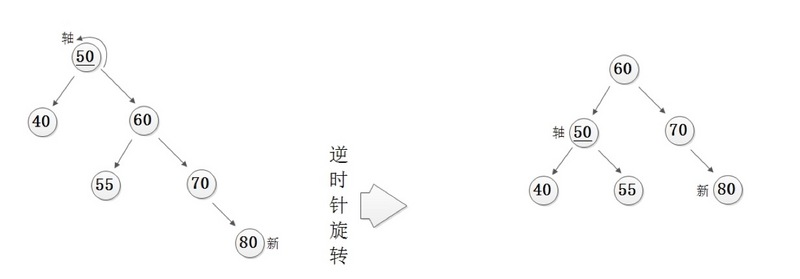/**
* 单旋 --- 左旋
*
* 适用情况：
*          1.新插入节点是右子树的右子节点
*          2.右子树的右子节点高度 >= 右子树左子节点的高度
* @return
*/
private AvlNode leftBalance(AvlNode root){
//左旋掕右左挂右
AvlNode node = root.right;
root.right = node.left;
node.left = root;

//高度重置
root.hight = Math.max(hight(root.left),hight(root.right))+1;
node.hight = Math.max(hight(node.right),hight(root))+1;

return node;
}

右旋
当新插入的节点是左子树的左子节点时，需要通过右旋来保持此部分子树继续保持平衡状态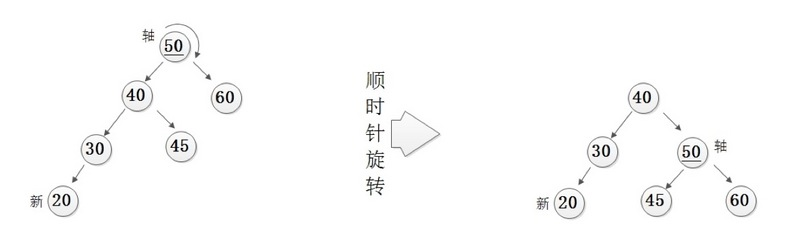/**
* 单旋 --- 右旋
*
* 使用情况：
*          1.新插入节点是左子树的左子节点
*          2.左子树的左子节点的高度 >= 左子树右子节点的高度
*
* @param root
* @return
*/
private AvlNode rightBalance(AvlNode root){
//右旋掕左右挂左
AvlNode node = root.left;
root.left = node.right;
node.right = root;

//高度重置
root.hight = Math.max(hight(root.left),hight(root.right))+1;
node.hight = Math.max(hight(node.left),hight(root))+1;

return node;
}

双旋

在某些情况下，我们需要旋转两次来保持树结构的平衡

先左旋再右旋
当新插入的节点是左子树的右子节点时，需要通过先左旋在右旋来保持此结构的平衡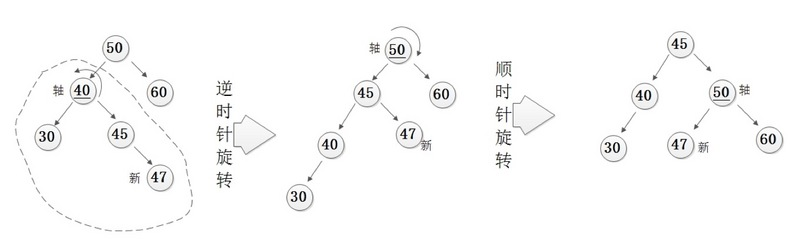/**
* 双旋 --- 先左旋后右旋
*
* 适用情况：
*         1.新插入节点是左子树的右子节点
*         2.左子树左子节点高度 <= 左子树右子节点高度
* @param root
* @return
*/
private AvlNode doubleLeftThenRight(AvlNode root){
//左旋
root.left = leftBalance(root.left);
//右旋
return rightBalance(root);
}

先右旋在左旋
当新插入的节点是右子树的左子节点时，需要通过先右旋在左旋来保持树的平衡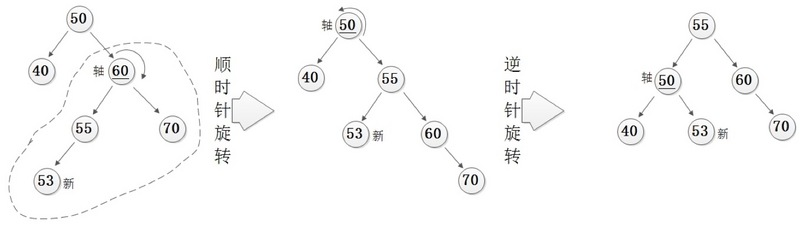/**
* 双旋 --- 先右旋后左旋
*
* 适用情况：
*          1.新插入节点是右子树的左子节点
*          2.右子树的左子节点高度 >= 右子树右子节点高度
* @param root
* @return
*/
private AvlNode doubleRightThenLeft(AvlNode root){
//右旋
root.right = rightBalance(root.right);
//左旋
return leftBalance(root);
}

依靠左旋和右旋来保持树的平和结构
平衡树结构
    /**
* 平衡树结构
* @param root
*/
private AvlNode balance(AvlNode root) {
if(root == null){
return root;
}
//新节点插入的左子树
if (hight(root.left) - hight(root.right) > 1){
//新节点插入的左子树的左子节点
if(hight(root.left.left) >= hight(root.left.right)){
//右旋
root = rightBalance(root);
}else{
//新节点插入的是左子树的右子节点
//先左旋后右旋
root = doubleLeftThenRight(root);
}
}
//新节点插入的右子树
else
if(hight(root.right) - hight(root.left) > 1){
//新节点插入的是右子树的右子节点
if(hight(root.right.right) >= hight(root.right.left)){
//左旋
root = leftBalance(root);
}else{
//新节点插入的右子树的左子节点
//先右旋后左旋
root = doubleRightThenLeft(root);
}
}
//高度重置
root.hight = Math.max(hight(root.left),hight(root.right))+1;
return root;
}

4.构造平衡树
构造平衡树的方法和构造二叉查找树一样，只是多了平衡结构建立而已
    /**
* 构建平衡树
* @param x
* @param root
* @return
*/
public AvlNode insert(int x,AvlNode root){
if(root == null){
return new AvlNode(null,null,x);
}
if(x > root.val){
root.right = insert(x,root.right);
}else if(x < root.val){
root.left = insert(x,root.left);
}else{
root.val = x;
}
return balance(root);
}

5.删除平衡树中指定节点
删除节点和二叉查找树一致，但是删除后，需要恢复平衡结构
    /**
* 删除平衡树中的指定节点
* @param x
* @param root
* @return
*/
public AvlNode remove(int x,AvlNode root){
if(root == null){
return root;
}
//寻找指定节点
if(x > root.val){
root.right = remove(x,root.right);
}else if(x < root.val){
root.left = remove(x,root.left);
}else{
//找到了指定节点
//判断是否存在子节点，以及是单个子节点还是两个子节点
if(root.left != null && root.right != null){
//左右子节点都存在
//找出右子树中的最小值
root.val = findMin(root.right).val;
//转成一个子节点的情况
root.right = remove(root.val,root.right);
}else{
//存在一个子节点,不空则覆盖
if(root.left != null){
root = root.left;
}
else if(root.right != null){
root = root.right;
}
//叶节点情况
else {
root = null;
}
}
}
//重新平衡
return balance(root);
}



展开全文...